# Test: CAT Quantitative Aptitude- 5

## 34 Questions MCQ Test CAT Mock Test Series | Test: CAT Quantitative Aptitude- 5

Description
Attempt Test: CAT Quantitative Aptitude- 5 | 34 questions in 40 minutes | Mock test for CAT preparation | Free important questions MCQ to study CAT Mock Test Series for CAT Exam | Download free PDF with solutions
QUESTION: 1

### Atish and Satish had a certain number of chocolates with them, not necessarily the same. Atish gave 80% of his total chocolates to Satish. After that, Satish gave 80% of the chocolates he now had to Atish. This process of Atish giving 80% of his chocolates to Satish and then Satish giving 80% of his chocolates back to Atish is considered one transaction. The ratio of the number of chocolates with Atish and Satish after each such transaction remains the same. What was the ratio of the total number of chocolates with Atish and Satish initially?

Solution:

Let the total number of chocolates that Atish and Satish initially had be 25a and 25b respectively.

After Atish gives 80% of his chocolates to Satish,
Chocolates with Atish = 5a
Chocolates with Satish = 20a + 25b
After Satish gives 80% of his chocolates to Atish,
Chocolates with Atish = 21a + 20b
Chocolates with Satish = 4a + 5b
Now,
21a + 20b = 25a
a / b = 5 /1
Initial ratio of the number of chocolates with Atish and Satish = 25a : 25b
= 5 : 1
Hence, option 4

QUESTION: 2

### If the interest compounded annually on a certain sum at a certain rate of interest for 2 years is equal to 40% of the simple interest on the same amount at same interest rate for thrice the time period, find the rate percentage.

Solution:

Let P be the sum and r be the rate of interest (expressed as a decimal fraction).
We have,
P (1 + r)2 - P = 0.40(6Pr)
P (1 + 2r + r2 - 1) = 2.4Pr
2r + r2 = 2.4r
r2 = 0.4r
r = 0.4 i.e., 40%
Hence, option 3.

QUESTION: 3

### Jagan, the video dealer, lends at most 10 DVDs at the cost of Rs. 300 per day and 12 CDs at the cost of Rs. 200 per day. Direct costs are Rs. 50 per day for DVDs and Rs. 30 per day for CDs. Also because some of the customers mishandled the products he had to spend Rs. 5000 on maintenance. In the month of March due to exams DVDs and CDs lending rate was 30% and 40% respectively, which kept on increasing gradually by 5% and 8% every month (i.e. rate became 35% and 48% respectively the next month). What is the profit for the month of May if his fixed monthly cost is Rs. 40,000?

Solution:

Fixed monthly cost = Rs. 40,000
First we need to compute the Total Revenue.
Revenue made by lending 10 DVDs in May = 31 x 10 x 300 = Rs. 93,000
Revenue made by lending 12 CDs in May = 31 x 12 x 200 = Rs. 74,400
But then the lending rate in May is 40% (it is gradually increasing by 5%)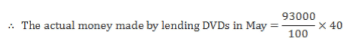= Rs. 37,200
Similarly, the lending rate in May is 56% (it is gradually increasing by 8%)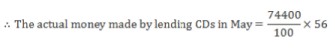= Rs. 41,664
Total direct cost of a DVD = 50x31 x 10 = Rs. 15,500
Total direct cost of a CD = 30 x 31 x 12 = Rs. 11,160
Maintenance cost = Rs.5,000
The revenue earned from all these products = (Actual money made by lending DVDs and CDs) - (direct cost of DVDs and CDs) - (Maintenance)
= Rs. 37,200 + Rs. 41,664 - Rs. 15,500 - Rs. 11,160 - Rs. 5,000
= Rs. 47,204
Profit or loss = revenue - expenses
= Rs. 47 ,204 - Rs . 40,000
= Rs. 7,204
This is positive that means his revenue is more than expense.
We have a profit of Rs. 7,204.
Hence, option 2.

*Answer can only contain numeric values
QUESTION: 4

Mr. Baker runs a bakery shop. One day he made some cakes of different weights. The weight of the heaviest two was 25% of the total weight of all the cakes and the weight of the lightest 5 cakes was 45% the total weight of all cakes. By the end of the day, he was able to sell the 5 lightest cakes. He took the two heaviest cakes home and closed the shop. Next morning he found that all the remaining cakes, which were of same weight, were stolen. How many cakes were stolen?

Solution:

Let the total weight of all the cakes be W.
The weight of the heaviest two cakes = 0.25 W
The average weight of each of the heaviest cakes = 0.25W/2 = 0.125 W
The weight of the lightest five cakes = 0.45 W
The average weight of each of the lightest cakes = 0.45 W/5 = 0.09 W
The weight o f the stolen cakes = W - 0.25 W - 0.45 W = 0.3 W
Let the number of stolen cakes be n.
0.09W < 0.3 W/n < 0.125 W
The only natural number that satisfies this condition is n = 3.

QUESTION: 5

Ram sells three articles A, B and C at profit of 10%, 20% and 30%, respectively. If the total profit he earns is 25% of the total cost, then how many of the following statements will necessarily hold true?

i. A was the least expensive article.
ii. B was the most expensive article.
iii. C was the most expensive article.
iv. B was the least expensive article.

Solution:

Let the cost prices of the three articles A, B and C are jc, y and z, respectively.
We have,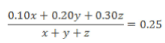Simplifying, we get:
z = y + 3x
Therefore, C was the most expensive of the three articles.
Therefore, statement 3 is necessarily true.
Therefore, statement 2 is incorrect.
The other statements may or may not be true.
Hence, option 2.

QUESTION: 6

A group of 10 students started playing pass-the-bucket. They stood in a row and the 1st student passed a bucket with 10 litres of milk. While passing it to the 2nd student in the row he spilled 5/3 litres. The 2nd student compensated for the lost volume by adding same amount of water and spilled 5/3 litres while passing the bucket to the 3rd student. This continued till the bucket was passed to the 10th student who replaced the volume of the spilled solution with an equal volume of water. What was the percentage of milk in the bucket when it was in the hands of the 5th student just before he handed it over to the 6th student?

Solution:

When the bucket reached the 5th student, 5/3rd solution in the bucket has been replaced by water 4 times.
Also, when the bucket reaches the 5th student 5/3rd of the solution in the bucket has been spilled.
After 4 replacements, amount of milk in solution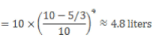When the bucket is passed to the 5th student 5/3 litres of the solution is spilled.
1/6th of the solution is spilled.
1/6th of the milk is spilled.
As equal percentages of milk and water are spilled, the percentage of milk in the bucket is
4.8/10 x 100 = 48%
Percentage of milk in the bucket when it was passed to the 5th student = 48%
Hence, option 3.

*Answer can only contain numeric values
QUESTION: 7

Robin has a silver ball and a platinum ball each of radii 4 cm. The worth of each ball is Rs. 50,000. The worth of silver doubles every 2 years, while that of platinum remains the same. At the end of 6 years, Robin sells the silver ball and invests the money in a new platinum ball. The worth of a platinum ball is directly proportional to its volume. How much should the radius (in cm) of the new platinum ball be?

Solution:

The initial worth of the ball was Rs. 50,000. The worth of silver doubles every 2 years.
Worth of the silver ball at the end of 6 years = Rs. (50,000 x 8) = Rs. 4,00,000
Robin invests Rs. 4,00,000 in a new platinum ball and the worth of a platinum ball is directly proportional to its volume.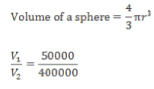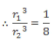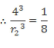r2 = 8 cm

QUESTION: 8

A tortoise and a hare run an 800 metre race, where the tortoise beats the hare by 100 metres. To do a favour to the disappointed hare, the tortoise starts 100 metres behind the starting line in a second 800 metre race. Both animals run at their earlier speeds. Which of the following is true in connection with the second race?

Solution:

When the tortoise completes 800 metres, the hare only completes 700 metres.
The ratio of the speeds of the tortoise and the hare is 8 : 7.
The two animals run the second race with the same speed, the ratio of the distances covered by the tortoise and the hare in the second race will also be 8 : 7.
When the tortoise covers 900 metres, the hare will cover 7/8 x 900 = 787.5 metres
The hare is beaten by the tortoise by 12.5 metres in the second race.
Hence, option 3.

*Answer can only contain numeric values
QUESTION: 9

Samrat covers the total journey of 810 km to his village partly by train and then by bus. The train is 20% faster than the bus. If the train were to travel for the number of hours, which normally would be hours travelled by bus, and the bus were to travel the remaining distance, then the time for the journey would reduce by 24 minutes. The normal travelling hours of bus and train are in the ratio 3:2. What is the speed(in km/hr) of the bus?

Solution:

Let the speed of the bus be b km/hr
The speed of the train = 12b km/hr
Let the bus normally travel for 3x hours and let the train normally travel for 2x hours.
3xb + 2.4xb = 810
xb = 150
If the train travels for the number of hours that the bus travels,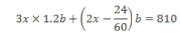5.6xb - 810 = 0.4b
5.6(150) -810 = 0.4b
b = 75
The speed of the bus = 75 km/hr

*Answer can only contain numeric values
QUESTION: 10

If 3x + 12 is perfectly divisible by a positive integer x, then the number of possible values of x is

Solution:

If 3x + 12 is perfectly divisible by x,

then (3x + 12)/x has to be an integer
i.e. 3 + 12/x has to be an integer
∴ 12/x is an integer.

x should be a divisor of 12, that is 1,2, 3, 4, 6, or 12.
Thus, x can have any of 6 possible values.

*Answer can only contain numeric values
QUESTION: 11

What is the number of solutions of the equation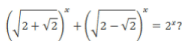Solution: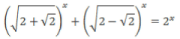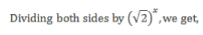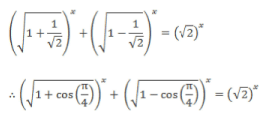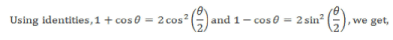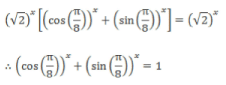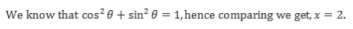Only one solution is possible.

QUESTION: 12

log212 is equal to

I. 2 + log2 3
II. log2 4 + log2 3 - 1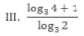Solution: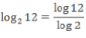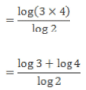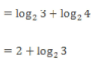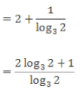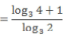Hence, option 2.

QUESTION: 13

If x3 - x - 1 = 0, then (x - 1) is equal to

Solution:

Consider the equation, x3 - x - 1 = 0,
x3 = x + 1 ...(i)
On multiplying the equation by x and x2 to get,
x4 = x2 + x . . . (ii)
x5 = x3 + x2 . ..(iii)
x5 = x3 + x2 = (x + 1) + x2 ... [Using (i)]
= (x + x2) + 1
= x4 + 1 ... [Using (ii)]

x5 - x4 = 1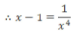Hence, option 4.

*Answer can only contain numeric values
QUESTION: 14

How many three digit numbers exist such that their value is decreased by 99% when their digits are reversed?

Solution:

The value of the three digit number decreases by 99% when its digits are reversed, we can see that the number with reversed digits must be 0.01 times the original three digit number.
The number formed on reversal of the digits must be a single digit number
(The number thus formed would become more than three digits on multiplication by 100).
The last two digits of the original three digit number must be zero, and the first digit must be a nonzero digit.
There are 9 such numbers: 100, 200, 300,..., 900.

QUESTION: 15

A wire of length 10 m is divided into two parts such that when each of the two parts is bent to form a square, the sum of the areas of the squares is 5 sq. m. What are the lengths of the two parts?

Solution:

Let x and 10 - x be the lengths of the two parts.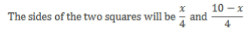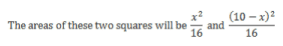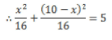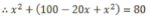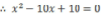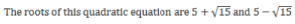Hence, option 4.

QUESTION: 16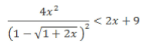Solution:

We have 1 + 2x > 0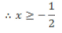Also we have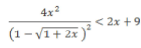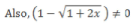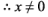Consider the given inequality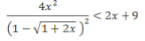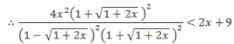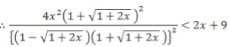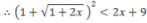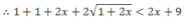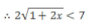4(1 + 2x) < 49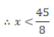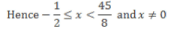Hence, option 3.

*Answer can only contain numeric values
QUESTION: 17

K is a set of even natural numbers. What is the largest natural number which will completely divide the product of any 3 consecutive numbers from the set K ?

Solution:

Here, K is the set of even natural numbers.
K = {2, 4, 6, 8,...}
For the maximum natural number which will always divide 3 consecutive numbers from set K i.e. 3 consecutive even natural number, we take lowest possible 3 numbers from set K, i.e. 2, 4 and 6.
We can easily recognise that 2 x 4 x 6 = 48 is the maximum natural number which completely divides 3 consecutive numbers from the set K.

Alternatively,

3 consecutive numbers from the set K (which is a set of even numbers) can be represented as 2n - 2, 2n, 2n + 2.
Their product = 2 x 2 x 2 x (n - 1) x n x (n + 1) = 8 x (n - 1) x n x (n + 1)
Now, (n - 1), n and (n + 1) are 3 consecutive natural numbers, so at least one of them must be even and at least one must be divisible by 3.
(n - 1) x n x (n + 1) will be definitely divisible by 6.
The product of the 3 consecutive numbers from set K must be definitely divisible by 8 x 6 = 48

*Answer can only contain numeric values
QUESTION: 18

Given a cube, I pick three random points from among its vertices. Let N be the number of ways in which three points can be selected such that they form a right angled triangle. What is N?

Solution:

The number of ways in which three points can be selected out of the eight vertices of a cube is 8C3 = 56
We can see that in most of these 56 ways, the three points selected form a right angled triangle. So, we look at possible selections of three points which would not give a right angled triangle.
We can see that if two of these three points are the endpoints of one of the edges of the cube, then irrespective of which of the remaining vertices is the third point, we always get a right angle. If two of the three points are endpoints of a body diagonal of the cube, then the third point will always be such that it will form an edge of the cube with one of the two points, so we again get a right angle.
So, the sides of the triangle formed by the three points should neither be edges nor the body diagonals of the cube, i.e. all three sides should be face diagonals.
To count the number of such triangles, consider the 'top' face ABCD of a cube ABCDWXYZ (the points corresponding to A, B, C and D on the bottom face are W, X, Y and Z respectively). It has two face diagonals, AC and BD. With AC as one of the sides, we can have two acute triangles, ΔACX and ΔACZ. With BD as one of the sides, we can have two acute triangles, ΔBDY and ΔBDW.
Similarly, WXYZ has two face diagonals, WY and XZ, from which we get four acute triangles, ΔBWY, ΔDWY, ΔAXZ and ΔCXZ. Thus, 8 triangles out of 56 are not right angled triangles.
56 - 8 = 48

QUESTION: 19

A circle with centre O has radius 12 cm. AD is the diameter and AB is the tangent to the circle at point A.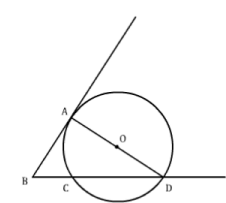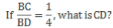Solution:

Let BC = x, BD = 4x
CD = BD - BC = 3x
Also AB2 = BC x BD = 4x2
AB = 2x
Now , in right angled  ΔABD
(4x)2 = (2x)2 + (24)2
16x2 = 4x2 + (24)2
12x2 = (24)2
x2 = 48 = 16 x 3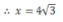CD = 3x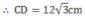Hence, option 3.

QUESTION: 20

If a, b and c are the sides of a triangle, then which of the following is true?

Solution:

a3 + b3 + c3 - 3abc = (a + b + c)(a2 + b2 + c2 - ab - be - ca)
In the above identity if we replace c by -c, we get,
a3 + b3 + c3 - 3abc = (a + b - c)(a2 + b2 + c2 - ab + bc + ca) ... (i)
By triangle inequality we know that
a + b> c or (a + b - c) > 0 ...(ii)
Also we can write,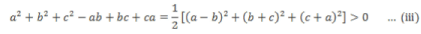Combining (ii) and (iii) and replacing in (i), we get,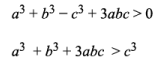Hence, option 4.

QUESTION: 21

If the area of a polygon is known, and the sum of squares of the diagonals is equal to the sum of squares of the sides of the polygon. What is the ratio of circumference of circumcircle and incircle drawn to it?

Solution:

Explanation : Sum of squares of the diagonals is equal to the sum of squares of the sides, which is true in case of a parallelogram.

This polygon has to be a parallelogram.

Sum of any two adjacent angles is 180°

Consider an incircle being drawn inside a parallelogram.

Tangents drawn from an external point to any circle are equal and opposite sides of a parallelogram are equal.

The parallelogram is a rhombus or a square.

But since all the angles of the quadrilateral are 90°

Therefore, Ratio of circumcircle to inradius is 1 : (2)1/2

QUESTION: 22

The difference in the areas of circumscribing and inscribing circles of the region bounded by the curves y = -|x| + 12 andy = |x| + 4 will be:

Solution: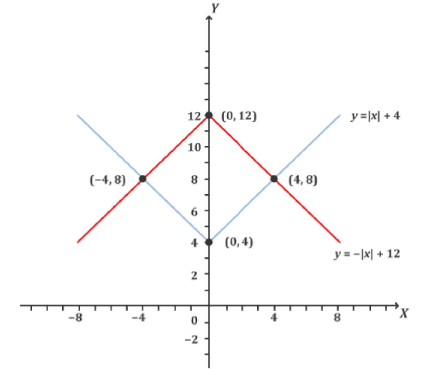As shown in the graph, we get the intersection points (-4, 8), (4, 8), (0, 12) and (0, 4).
The enclosed region would be a square with each side of length of 4√2
The radius of the inscribed circle = 2√2
It's area = 8π
The radius of the circle circumscribing the square will be 4.
It’s area = 16π
The difference between these two areas = 16π - 8π = 8π
Hence, option 2.

QUESTION: 23

If a = rcosθ then which of the following is tan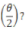Solution:

Let θ = 90°
cos90° = 0
a = 0
tan(θ/2) = tan45° = 1
Hence, substitute a = 0 in each option and identify the option where the numerator and denominator become equal.
Among the given options, option 2 satisfies this condition.
Hence, option 2.
Alternatively,

a - rcosθ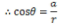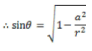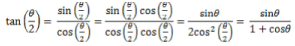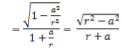Hence, option 2.

*Answer can only contain numeric values
QUESTION: 24

Suraj was relocating from Hyderabad to Kolkata. He had a spherical shape glass memento that he did not want to break during transportation. Therefore, he purchased a right circular cylinderical box made of steel costing Rs. 600 in which the glass memento exactly fitted. He then covered the cylinder with bubble wrap costing Rs. 2 per sq. cm. If the volume of the memento was (256/81) cm3 then what was the approximate total expenditure that Suraj incurred for protecting it?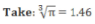Solution:

Let the radius of the memento be r.
The radius and height of the cylinder such that the spherical memento just fits in will be r and 2r cm.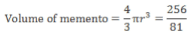r = 0.91
Total Surface area of the cylinder = 2πr(h + r) = 2πr(2r + r) = 6πr2
= 6 x 3.14x0.912
~ 15.6 cm2 Cost of the bubble wrap = 15.6 x 2 = Rs. 31.2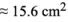Cost of the bubble wrap = 15.6 x 2 = Rs. 31.2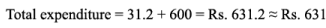QUESTION: 25

If we draw graphs of the following equations using standard conventions, for how many of the graphs will a line parallel to the y-axis intersect more than once?

i. xy = 4
ii. y = tan(x)
iii. x2 +y2 = 8
iv. x = y2
v. |x| + |y| = 2

Solution:

i.xy = 4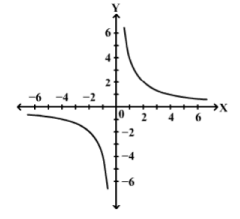∴ A line parallel to the y-axis can intersect the graph o f xy = 4 at only one point.
ii. y = tan(x)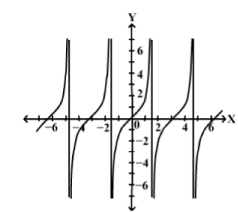∴ A line parallel to the y -axis can intersect the graph o f y = tan(x) at only one point.
iii. x2 + y2 = 4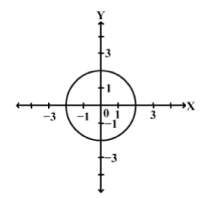∴ A line parallel to the y-axis can intersect the graph of x2 + y2 = 4 twice.
iv. x = y2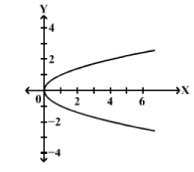∴ A line parallel to the y -a x is can intersect the graph o f x = y2 twice.

v. |x|+ |y| = 2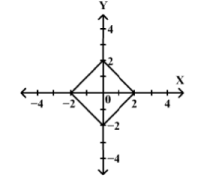∴ A line parallel to the 7-axis can intersect the graph of |x| + |y| = 2 twice.
Hence, option 3.
Alternatively,
A line parallel to the y-axis intersects the graph of an equation twice if for a given value of x, there can be two values of y.
Consider the given equations:
i. xy = 4
∴ y = 4/x
For a given value of x, y can take only one value.
A line parallel to the y-axis intersects the graph of xy = 4 only once.
ii. y = tan x
For a given value of x, y can take only one value.
iii. x2 + y2 = 8 .
∴ y2 = 8 - x2
Clearly, for a given value of x, y can take two values.
∴ A line parallel to the y-axis intersects the graph of x2 + y2 = 8 twice.
iv. x = y2
Again, here it is clear that for a given value of x, y can take two values.
v. |x| + [y| = 2
∴ |y| = 2 - |x|
For every value of |x|, y can take a positive and a negative value.
Thus we can see that a line parallel to the y-axis intersects the graphs of (iii), (iv) and (v) twice.
Hence, option 3.

QUESTION: 26

People who like chocolate and ice-cream is 25% of people who like only chocolate. People who like ice-cream are 66.66% of people who don’t like chocolate. People who like only ice-cream is 90% of people who don’t like ice-cream. What is the ratio of people who like both to none?

Solution:

Let people who:

like ice-cream only = Do not like chocolate = x
like chocolate only = Do not like ice-cream = y
like neither chocolate nor ice-cream = z
From conditions given in the question,
People who like both ice-cream and chocolate = 0.25y
x + 0.25y = 0.66(x + z) ...(i)
x = 0.9 (y + z) ... (ii)
Solving (i) and (ii),
y/z = 2/3
The ratio of people who like both to none = 0.25y : z = 1 : 6
Hence, option 2.

QUESTION: 27

The sum of all the terms of an infinite geometric progression is 30. The sum of the squares of all the terms of that progression is 300. What is the sum of the first two terms of the progression?

Solution:

In an infinite geometric progression, if a is the first term and r the common ratio, then the sum of all the terms is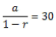The series formed by the squares is also a G.P., with a2 as the first term and r2 as the common ratio, so the sum is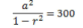Dividing the second equation by the first, we get,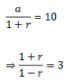∴ r = 0.5 and a = 15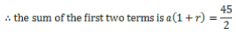Hence, option 2.

*Answer can only contain numeric values
QUESTION: 28

A student has to attempt exactly 10 questions out of 15 in an exam ensuring that at least 3 out of the first 5 questions are attempted. In how many ways can the questions be selected?

Solution:

Three questions are attempted from the first five.
These can be selected in 5C3 = 10 ways
Also the remaining 7 questions can be attempted from the next 10 in 10C7 = 120 ways
Therefore the total number of ways in which questions can be selected in the exam, if three questions are attempted from the first five questions, is 10 x 120 = 1200

Case II:

Four questions are attempted from the first five.
These can be attempted in 5C4 = 5 ways
Also the remaining 6 questions can be attempted from the next 10 in 10C6 = 210 ways
Therefore the total number of ways in which questions can be selected in the exam, if four questions are attempted from the first five questions, is 5 x 210 = 1050

Case III:

All five questions are attempted from the first five.
These can be attempted in 5C5 = 1 way
Also the remaining 5 questions can be attempted from the next 10 in 10C5 = 252 ways
Therefore the total number of ways in which questions can be selected in the exam, if five questions are attempted from the first five questions is 1 x 252 = 252
Therefore the total number of ways of selecting questions in the exam is 1200+ 1050 + 252 = 2502

QUESTION: 29

The probability that Ganguly plays and the team wins is 2/7 and the probability that the team wins is 6/7. What is the probability that Ganguly played when the team won?

Solution:

Let: x = Match in which Ganguly plays andy = Match in which the team wins
Given: P(x ∩ y) = 2/7, P(y) = 6/7
We need to calculate: P(x/y)
P(x/y) = P(x ∩ y)/P(y) = (2/7) x (7/6) = 1/3

Hence, option 4.

*Answer can only contain numeric values
QUESTION: 30

The arithmetic mean of a set of 20 observations is X. When three of the twenty observations, 12, 19 and 74 are discarded, the mean of the new set remains unchanged. What is the arithmetic mean, X?

Solution:

Sum of the original set of 20 observations = 20 x X

Sum of the new set of 17 observations = 17 x X
Now, the new set is formed by discarding three of the elements of the original set. 20X- (12 +19 + 74) = 17X
3X= 105
X = 35

Alternatively,

As the mean of the original set does not change when the three numbers are discarded, hence, the mean of the original set is same as the mean of the three discarded numbers.
The mean of the three discarded numbers is (19 + 74 + 12)/3 = 35
X= 35

QUESTION: 31

Group Question

Answer the following question based on the information given below.

In a company three projects were assigned to all the members of a company. Following information is also known: 12 members were selected for the entire three projects.
The ratio for number of members selected for only project A : only project B : only project C is 3 : 4 : 5.
The ratio for number of members selected for project B and C only : project C and A only : project A and B only is 2 : 3 : 4.

Q. If the ratio of number of members selected for project A and C only to the members selected for only project B is 7 : 20, find the ratio of the members selected for B and C only to the members selected for only C?

Solution:

Refer the Venn diagram,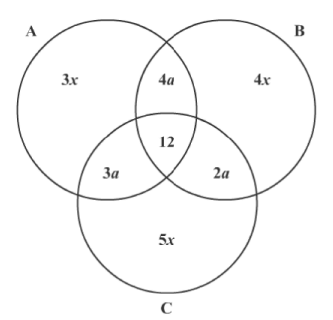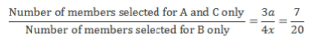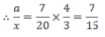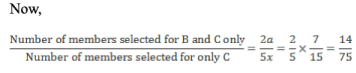Hence, option 2.

QUESTION: 32

In a company three projects were assigned to all the members of a company. Following information is also known: 12 members were selected for the entire three projects.
The ratio for number of members selected for only project A : only project B : only project C is 3 : 4 : 5.
The ratio for number of members selected for project B and C only : project C and A only : project A and B only is 2 : 3 : 4.

Q. If the ratio of sum of number of members for only A, only B, only C to the sum of members for A and B only, B and C only, A and C only is 20/7 and the ratio of sum of number of members of A and B only, B and C only, A  and C only to the members selected for all the three projects is 21/4, then find the number of members selected for only project C.

Solution:

According to the diagram in the previous question,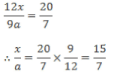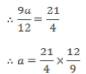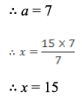∴ Number of members selected for only C = 5x = 15 x 5 = 75

Hence, option 4.

*Answer can only contain numeric values
QUESTION: 33

Group Question

Answer the following question based on the information given below.

A and B together can complete some work in 24 days. If A, B and C work together, they can complete the same work in 18 days. B and C both have the same efficiency.

Q. , B and C start working together from the very first day. If A works for 8 days, B works for 16 days and C works till the end of the given work, how many days are required to complete the given work?

Solution:

Let A require ‘a’ days, B require ‘b’ days and C require ‘c’ days to complete the work separately.
A and B together both can complete a work in 24 days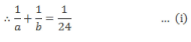A, B and C all three can complete a work in 18 days.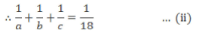Solving equation (i) and (ii), we get,
∴ c = 72 days
∴ C requires 72 days to complete the work separately.
So, B requires 72 days to complete the work separately.
Putting this value of b = 72 in equation (i), we get,
a = 36 days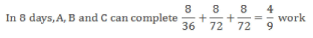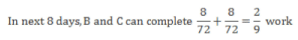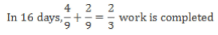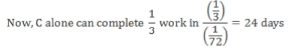∴ The number of days required to complete the work = 16 + 24 = 40 days
Alternatively,
Let the total amount of work to be done be the L.C.M. of 24 and 18 i.e. 72 units.

Let A, B and C complete a, b and c units of work per day respectively when working alone.
∴ a + b = 72/24 = 3 units/day
and a + b + c = 72/18 = 4 units/day
∴ c = 4 - 3 = 1 unit/day
∴  b =1 unit/day and a = 2 units/day
Now, A, B and C work together for the first 8 days.
In this time frame, they complete 8(a + b + c) = 8(1 + 1 + 2) = 32 units of work.
Then, B and C work together for the next 8 days.
So, in this period, they complete 8(b + c) = 8(1 + 1) = 16 units o f work.
Thus, by the time, C starts working alone, 32 + 16 = 48 units of work has been completed.
Now, C completes the remaining 24 units of work alone by completing 1 unit per day.
Thus, C takes 24 days to complete the remaining work alone.
So, total time taken = 8 + 8 + 24 = 40 days.

*Answer can only contain numeric values
QUESTION: 34

A and B together can complete some work in 24 days. If A, B and C work together, they can complete the same work in 18 days. B and C both have the same efficiency.

Q. A, B and C start working together from the very first day. If C leaves after 6 days and D (whose efficiency is equal to A) joins the work at that instant, then approximately how many days will be required to complete the work?

Solution:

We know that, A, B and C require 36, 72 and 72 days respectively to complete the work when working alone.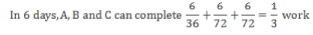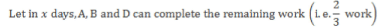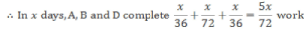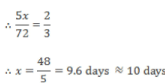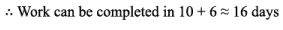From the solution to the previous question, total work = 72 units and the efficiency of A, B and C (in units/day) is 2, 1 and 1 respectively.
Since the efficiency of D is the same as that of A, d = 2 units/day as well.
A, B and C work together for the first 6 days.
So, amount of work done in the first 6 days = 6(a + b + c) = 6(2 + 1 + 1) = 24 units.
Now, C leaves and D joins the work.
So, the remaining 48 units of work is done by A, B and D.
Let the number of days taken by these three people by x.
So, 48 = (2 + 1 + 2) * x
x = 48/5 = 9.6 days
Since the work is incomplete at the end of day 9, the number of days taken has to be considered as 10.
So, total time taken to complete the work = 6 + 10 =16Use Code STAYHOME200 and get INR 200 additional OFF Use Coupon Code